# Samacheer Kalvi 12th Maths Solutions Chapter 1 Applications of Matrices and Determinants Ex 1.2

## Tamilnadu Samacheer Kalvi 12th Maths Solutions Chapter 1 Applications of Matrices and Determinants Ex 1.2

Question 1.
Find the rank of the following matrices by the minor method: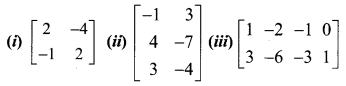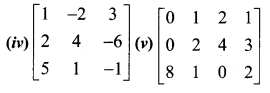Solution: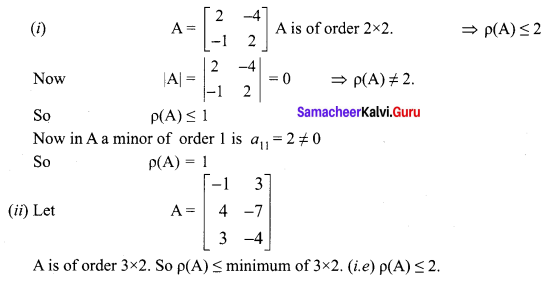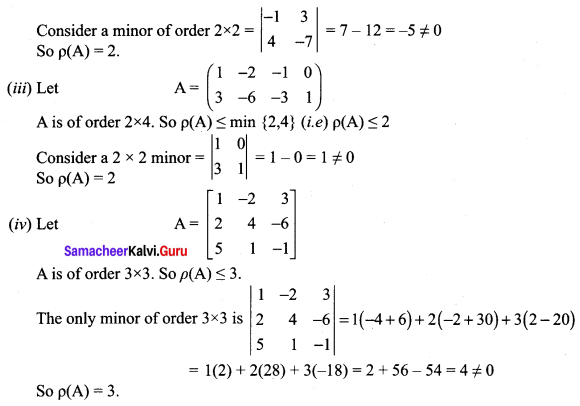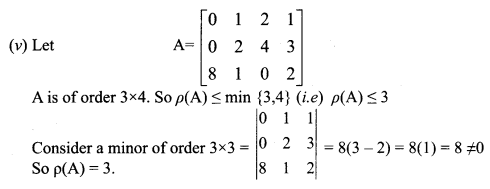Question 2.
Find the rank of the folowing matrices by row reduction method: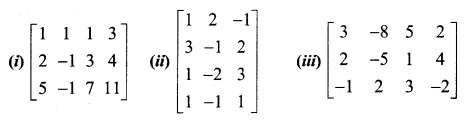Solution:
(i) Let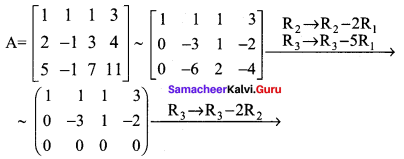The last equivalent matrix is in row-echelon form. It has three non zero rows. So ρ(A) = 3
(ii) Let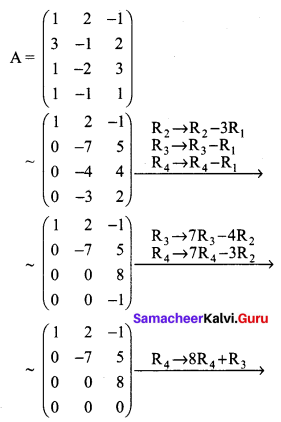The last equivalent matrix is in row-echelon form. It has three non zero rows. ρ(A) = 3
(iii) Let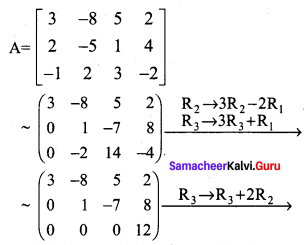The last equivalent matrix is in row-echelon form. It has three non zero rows. ρ(A) = 3

Question 3.
Find the inverse of each of the following by Gauss-Jordan method: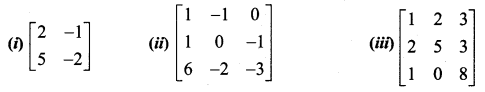Solution:
(i) Let $$A=\left(\begin{array}{cc}{2} & {-1} \\ {5} & {-2}\end{array}\right)$$
Applying Gauss-Jordan method we get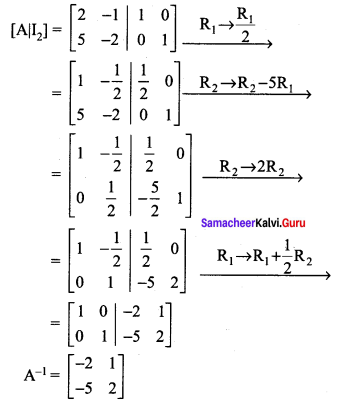(ii) Let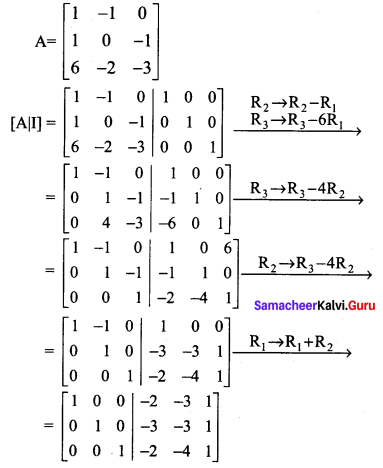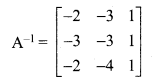(iii) Let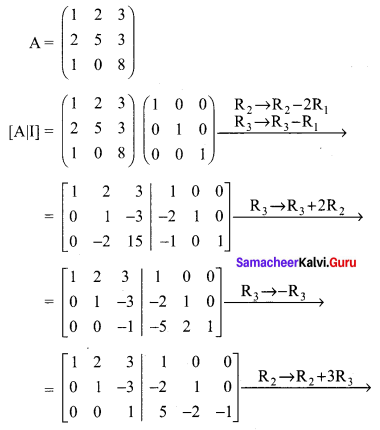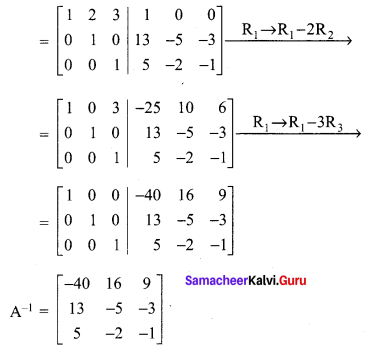### Samacheer Kalvi 12th Maths Solutions Chapter 1 Applications of Matrices and Determinants Ex 1.2 Additional Problems

Question 1.
Find the rank of the following matrices.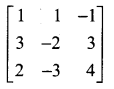Solution: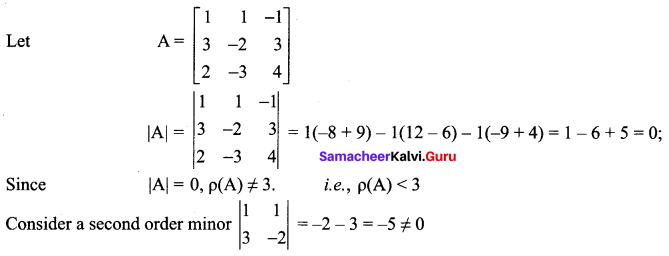A has at least one non-zero minor of order 2. $$\rho(\mathrm{A})$$ = 2

Question 2.
Find the rank of the following matrices.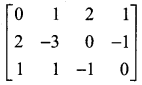Solution: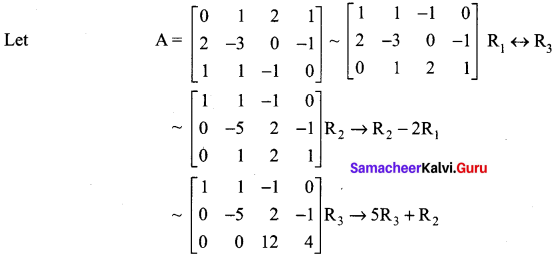The last equivalent matrix is in the echelon form. It has three non-zero rows.
∴ $$\rho(\mathrm{A})$$ = 3; Here A is of order 3 × 4

Question 3.
Find the rank of the following matrices.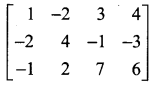Solution: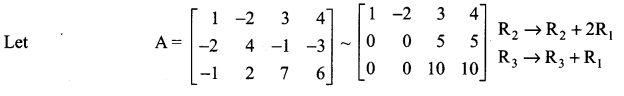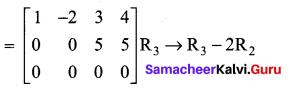The last equivalent matrix is in the echelon form. The number of non-zero rows in this matrix is two. A is a matrix of order 3 × 4. ∴ $$\rho(\mathrm{A})$$ = 2

Question 4.
Using elementary transformations find the inverse of the following matrix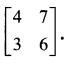Solution: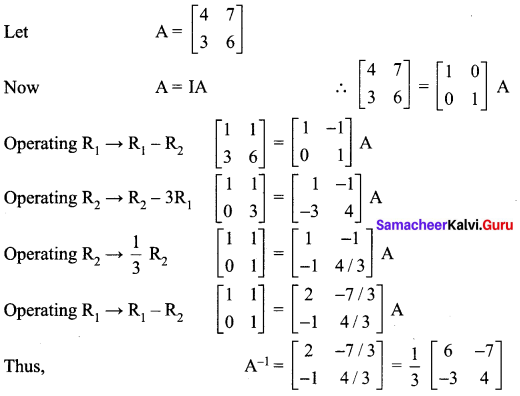Question 5.
Using elementary transformations find the inverse of the following matrices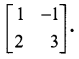Solution: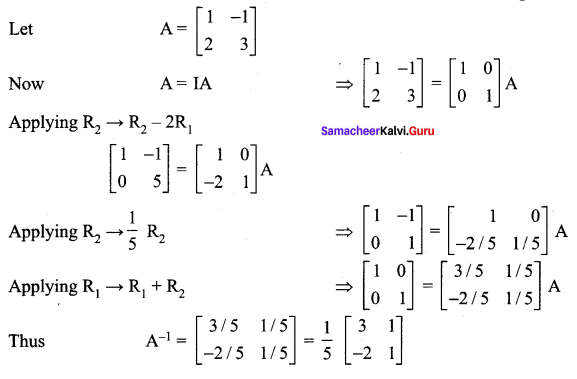Question 6.
Using elementary transformations find the inverse of the following matrices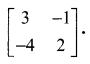Solution: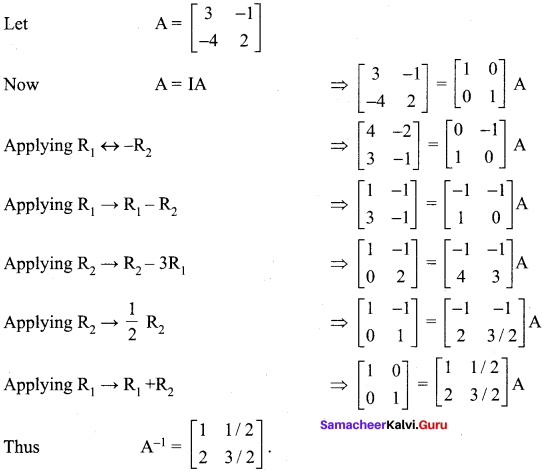Question 7.
Using elementary transformations, find the inverse of the following matrices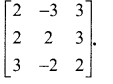Solution: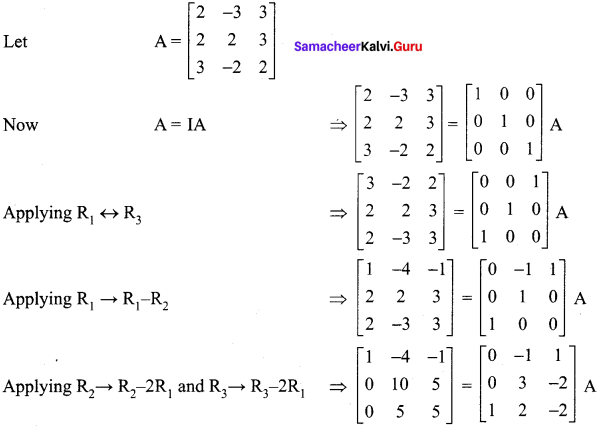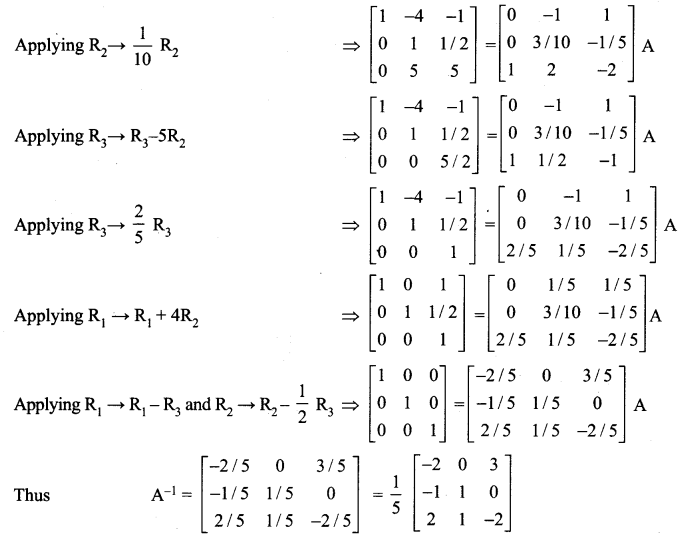Question 8.
Using elementary transformations, find the inverse of the following matrices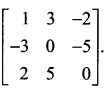Solution: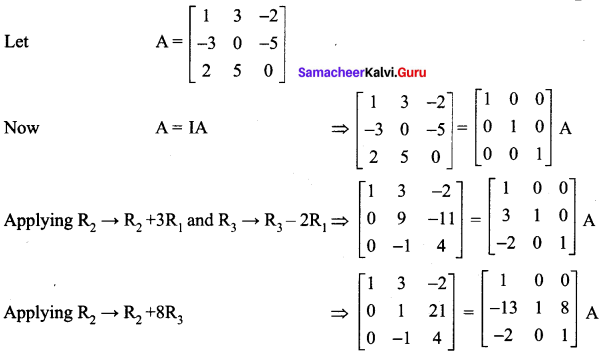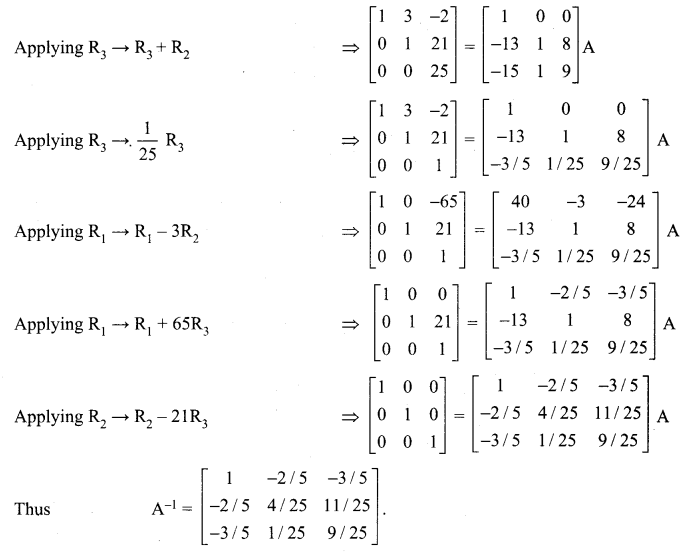Question 9.
Using elementary transformations, find the inverse of the following matrices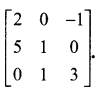Solution: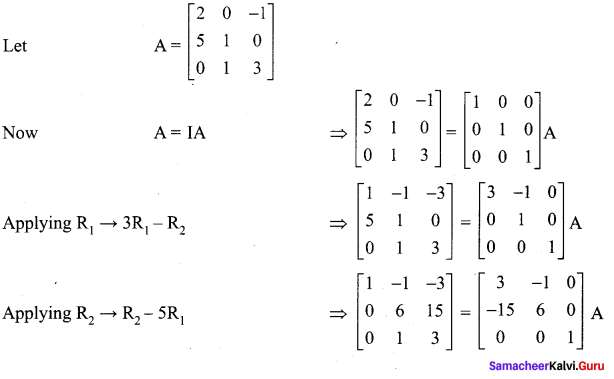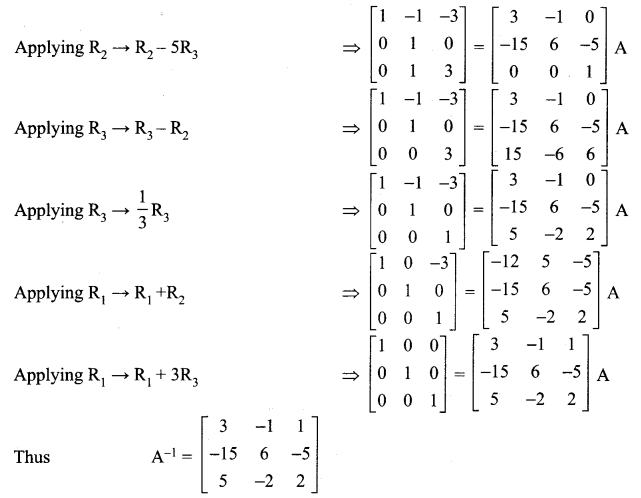Question 10.
Using elementary transformations, find the inverse of the following matrices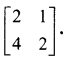Solution: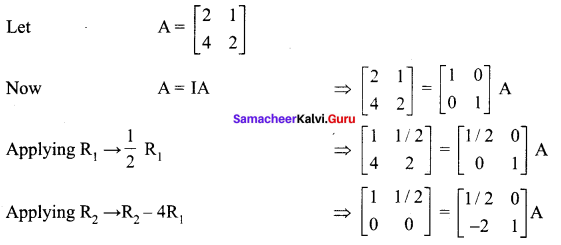Since R2 has all numbers zero, Thus inverse of matrix A does not exist.